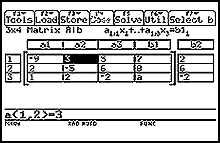Simultaneous Equation Solver App for the Voyage™ 200 and TI-92 PlusExtend the benefits and functions of your calculator. Calculate solutions (unique, representation of the infinite solutions, or no solution) for linear systems up to 30x30.

Simultaneous Equation Solver 1.00 83
Guidebooks
Simultaneous Equation Solver guidebook for TI-89 / TI-92 Plus / Voyage 200 (English) View: 135
Simultaneous Equation Solver guidebook for TI-89 / TI-92 Plus / Voyage 200 (Espanol) View: 144
Simultaneous Equation Solver guidebook for TI-89 / TI-92 Plus / Voyage 200 (Francais) View: 142

Features Summary

Extend the benefits and functions of your calculator. Calculate solutions (unique, representation of the infinite solutions, or no solution) for linear systems up to 30x30.